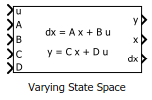Varying State Space

State-space model with varying matrix values

• Library:
• Control System Toolbox / Linear Parameter Varying

•Description

Use this block to implement a continuous-time state-space model with varying matrices. Feed the instantaneous values of the state matrix A, input matrix B, output matrix C, and feedforward matrix D to the corresponding input ports. The system response is given by:

$\begin{array}{c}dx=Ax+Bu\\ y=Cx+Du,\end{array}$

where u is the system input, y is the system output, and x and dx are the state vector and state derivatives, respectively.

Use this block and the other blocks in the Linear Parameter Varying library to implement common control elements with variable parameters or coefficients. For more information, see Model Gain-Scheduled Control Systems in Simulink.

Ports

Input

expand all

System input signal.

State matrix of dimensions Nx-by-Nx, where Nx is the number of system states.

Input matrix of dimensions Nx-by-Nu, where Nu is the number of system inputs.

Output matrix Ny-by-Nx, where Ny is the number of system outputs.

Feedforward matrix of dimensions Ny-by-Nu.

Output

expand all

System output signal.

Current state values.

Dependencies

To enable this port, select the Output states parameter.

Current derivatives of the corresponding states in x.

Dependencies

To enable this port, select the Output state derivatives parameter.

Parameters

expand all

Initial state values, specified as a scalar or a vector whose length is the number of system states.

To identify system states, specify state names as a:

• character vector, for a one-state plant.

• Cell array of character vectors, for a multistate plant.

Select to enable the state values output port, x.

Select to enable the state derivatives output port, dx.

Extended Capabilities

C/C++ Code GenerationGenerate C and C++ code using Simulink® Coder™.

Introduced in R2017b